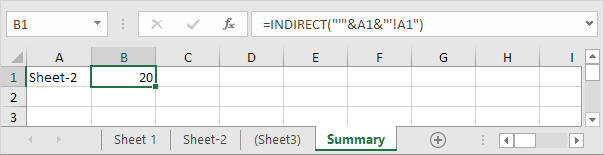# Indirect

Use the INDIRECT function in Excel to convert a text string into a valid reference. You can use the & operator to create text strings.

### Cell Reference

Use the INDIRECT function in Excel to convert a text string into a valid cell reference.

1. For example, take a look at the INDIRECT function below.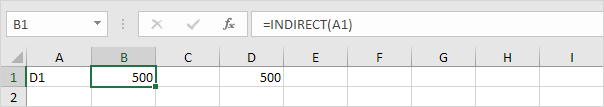Explanation: =INDIRECT(A1) reduces to =INDIRECT("D1"). The INDIRECT function converts the text string "D1" into a valid cell reference. In other words, =INDIRECT("D1") reduces to =D1.

2. The simple INDIRECT function below produces the exact same result.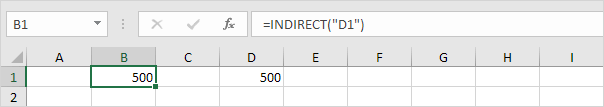3. Do we really need the INDIRECT function? Yes. Without using the INDIRECT function, this would be the result.4. Use the & operator to join the string "D" with the value in cell A1.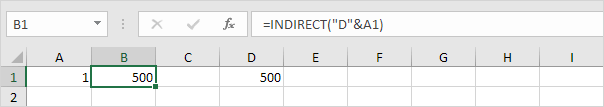Explanation: the formula above reduces to =INDIRECT("D1"). Again, =INDIRECT("D1") reduces to =D1.

### Range Reference

Use the INDIRECT function in Excel to convert a text string into a valid range reference. For example, use SUM and INDIRECT.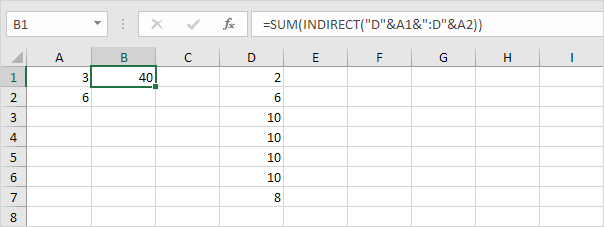Explanation: the formula above reduces to =SUM(INDIRECT("D3:D6")). The INDIRECT function converts the text string "D3:D6" into a valid range reference. In other words, =SUM(INDIRECT("D3:D6")) reduces to =SUM(D3:D6).

### Named Range

Use the INDIRECT function in Excel to convert a text string into a valid named range.

1. For example, the AVERAGE function below uses the named range Scores.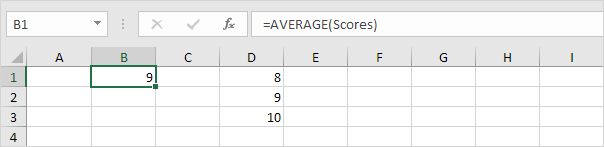Explanation: the named range Scores refers to the range D1:D3.

2. However, the AVERAGE function below returns an error.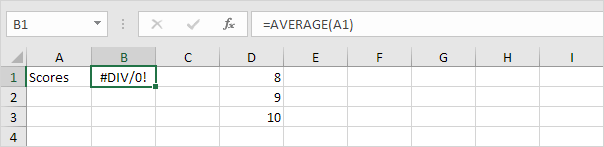Explanation: =AVERAGE("Scores") returns an error because Excel cannot calculate the average of a text string!

3. The INDIRECT function below does the trick.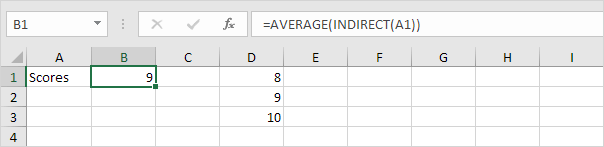Explanation: =AVERAGE(INDIRECT("Scores")) reduces to =AVERAGE(Scores).

### Worksheet Reference

Use the INDIRECT function in Excel to create a dynamic worksheet reference.

1. This is what a simple worksheet reference looks like.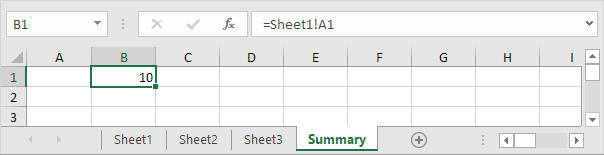Note: cell A1 on Sheet1 contains the value 10. Cell A1 on Sheet2 contains the value 20. Cell A1 on Sheet3 contains the value 30.

2. On the Summary sheet, enter the INDIRECT function shown below. Use the & operator to join the sheet name in cell A1 with "!A1".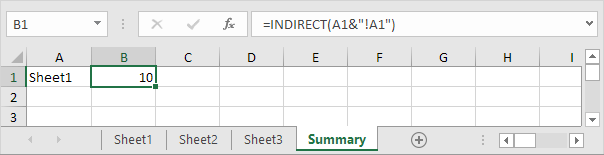Explanation: the formula above reduces to =INDIRECT("Sheet1!A1"). The INDIRECT function converts the text string "Sheet1!A1" into a valid worksheet reference. In other words, =INDIRECT("Sheet1!A1") reduces to =Sheet1!A1.

3. If your sheet names contain spaces or other special characters, enclose the sheet name in single quotation marks. Modify the INDIRECT function as shown below.